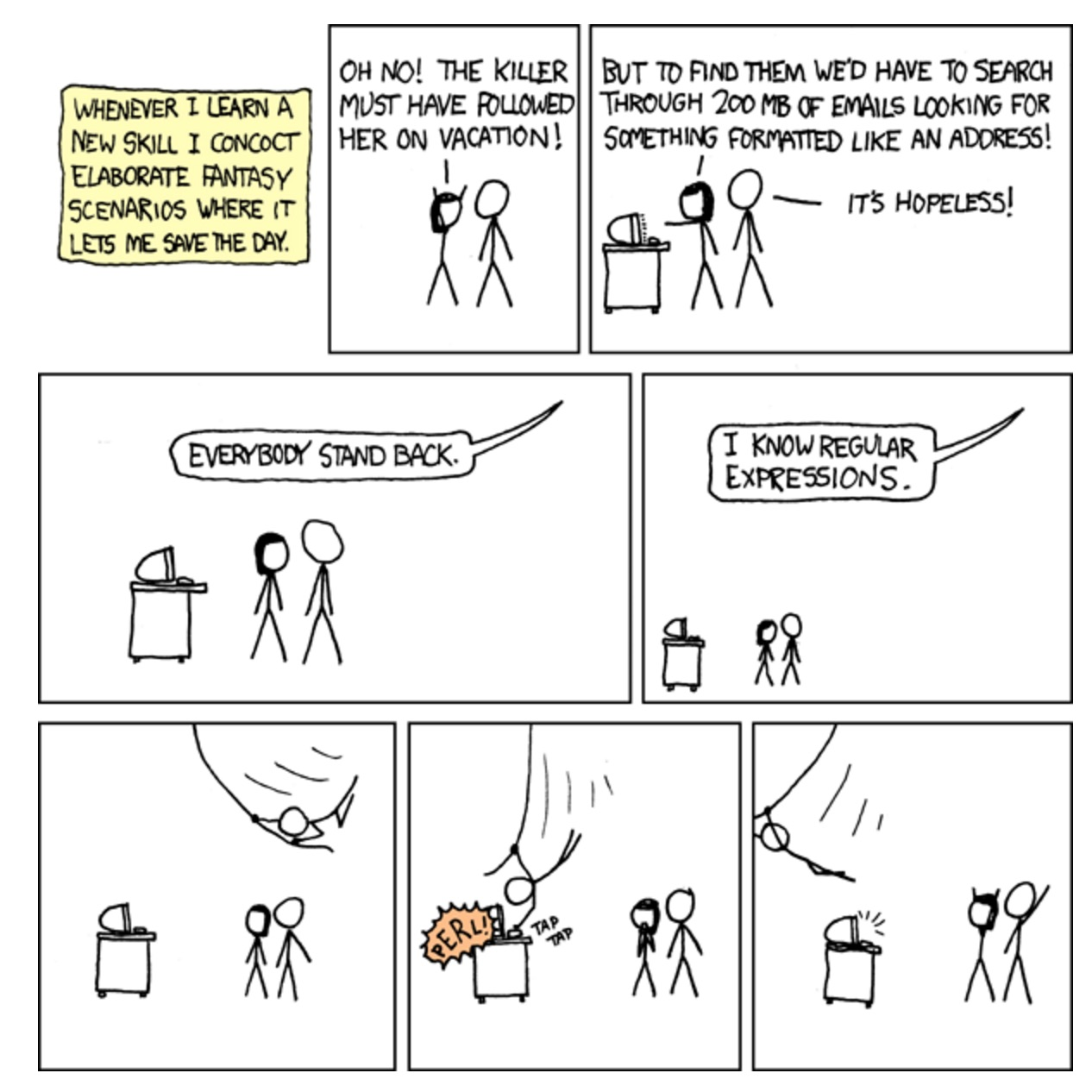# Acknowledgements

1. Live: The code is executed in your browser
2. Interactive: You can modify the code and it is evaluated as you type

In his repo, Mark shows how to use regular expressions and automatas to solve programming riddles. In this article, we will focus on regular expressions.# Prelude

For the purposes of this code, it is useful to replace Clojure’s `max` / `max-key` with versions that return `nil` when passed no inputs to maximize. Also, we are going to use clojure.combinatorics by Mark Engelberg for generating cartesian products of sequences:

``````
(ns my.combinatorics
(:require [clojure.math.combinatorics :refer [cartesian-product]]))

(defn max ([] nil) ([& s] (apply clojure.core/max s)))
(defn max-key ([k] nil) ([k & s] (apply clojure.core/max-key k s)))
``````

# The classic interview problem - maximum segment sum

A popular problem is to find an `O(n)` algorithm for computing the maximum sum achievable by adding up some contiguous subsequence (aka segment) of a sequence of numbers (typical input is a mix of positive and negative integers).

For example,`(maximum-segment-sum [-1 2 3 -4 5 -8 4])` should return `6` because `2+3+-4+5` is `6`.

If you’ve never seen this problem before, I encourage you to go try to solve it right now. It’s a fun problem.

The trick is to keep a running sum as you traverse the sequence, never letting the running sum dip below 0. This has the effect of ignoring negative numbers that make things “too negative”. Return the highest sum you saw.

This strategy can be implemented concisely in Clojure:

``````(defn maximum-segment-sum [s]
(apply max (reductions (comp #(max 0 %) +) 0 s)))
``````

Let’s the results of the `reductions` with `[-1 2 3 -4 5 -8 4]`:

`````` (reductions (comp #(max 0 %) +) 0 [-1 2 3 -4 5 -8 4])
``````

The max is `6`.

# A harder problem - maximum non-segment sum

But we’re going to do something harder, we’re looking for the maximum sum among subsequences that are not a continguous segment.

For example, `(maximum-non-segment-sum [-1 4 5 -3 -4])` should be `5` because `4+5+-4 = 5`, and those three numbers don’t form a segment.

We can’t choose just `4`, or just `5`, or `4+5`, because singletons and adjacent pairs are considered a segment. We can’t even choose the “empty” subsequence with a value of `0`, because that is also considered a segment. We could have chosen things like `-1+5` or `5+-4` or `4+-3`, but they happen to be not as good.

Unfortunately, there’s no clever trick for solving this problem. We’re going to have to look for a more principled approach.

(If you don’t believe me, go spend a while trying to solve it, just so you can appreciate how hard this problem really is.)

# Brute force with Regular expressions

Our strategy is going to be brute force:

``````(defn maximum-non-segment-sum [s]
(->> (all-non-segment-subsequences s)
(map (partial apply +))
(apply max)))
``````

But how to write `all-non-segment-subsequences`?

First key insight is that you can represent a subsequence by applying a bitmask of `0`s and `1`s to the sequence.

``````(defn apply-bitmask
"Takes a sequence and a bitmask, and returns the correpsonding subsequence"
(for [[item bit] (map vector s bitmask) :when (= bit 1)] item))
``````

Let’s see how it works:

``````  (apply-bitmask [1 2 3 4 5] [0 1 1 0 1])
``````

We can describe the satisfactory bitmasks with a regex

``````(def non-segment-regex #"0*1+0+1(0|1)*")
``````

What this regex says is that a non segment bitmask is a sequence of:

• 0 or `0`s
• 1 or more `1`s
• 1 or more `0`s
• a single `1`
• `0`s or `1`s freely

And indeed, this regex recognizes whether a bitmask represents a non-segment

``````(re-matches non-segment-regex "011010" )
``````

or not

``````(re-matches non-segment-regex "011110")
``````

``````(defn non-segment-bitmask?
"Takes a sequence of 0's and 1's and determines whether this represents a subsequence that is not a contiguous segment"
[s]
(not (nil? (re-matches non-segment-regex (clojure.string/join s)))))
``````

It works as expected:

``````(map non-segment-bitmask? [[0 1 1 1] [0 1 1 1 0 1]])
``````

Now, we are ready to write our `all-non-segment-subsequences`: we will generate all the `0`s and `1`s sequences of the desired length and `filter` with `non-segment-bitmask?`.

We will use the `cartesian-product` from `clojure.combinatorics`:

``````(defn all-non-segment-subsequences
"Takes a sequence and returns all subsequences that are not a contiguous segment"
[s]
(->> (apply cartesian-product (repeat (count s) [0 1])) ; all bitmasks matching s's length
``````

Let’s take a look at all the non-segment subsequences of `[1 2 -3 4 -5]`:

``````(all-non-segment-subsequences [1 2 -3 4 -5])
``````

And now, all the pieces of the puzzle are in place in order to run the `maximum-non-segment-sum` that we wrote above:

``````(maximum-non-segment-sum [-1 4 5 -3 -4])
``````
``````(maximum-non-segment-sum [-1 4 5 -3 4 -9 10])
``````

Please don’t try to run it with too long sequences!

(On my browser it starts to take too much time with 15 elements).

In our next article, we will show how to make our algorithm much more efficient using automatas.